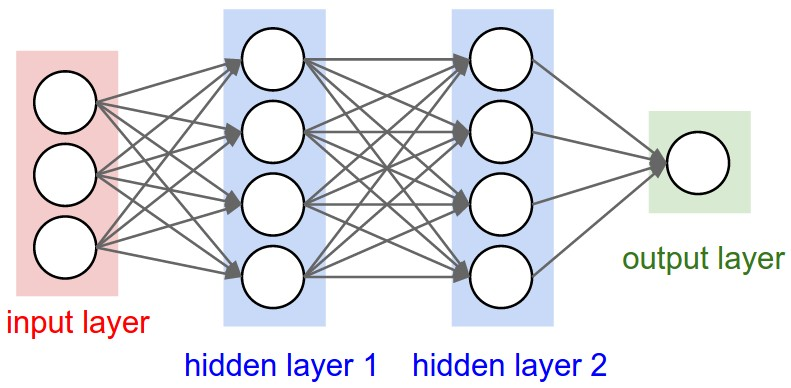# Measuring the Size of Neural Networks

December 27, 2017

Neural networks are sized in one of two ways: the number of neurons, or the number of trainable parameters. When given the following two neural networks:We can either say that this 2-layer network has $$4 + 2 = 6$$ neurons (don’t include the inputs), or that the network has $$3 * 4 + 4 * 2 = 20$$ weights and $$4 + 2 = 6$$ biases for a total of 26 learnable parameters.In this 3-layer network, we see $$4 + 4 + 1 = 9$$ neurons or $$3 * 4 + 4 * 4 + 4 * 1 = 32$$ weights and $$4 + 4 + 1 = 9$$ biases, for a total of 41 learnable parameters.